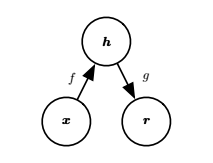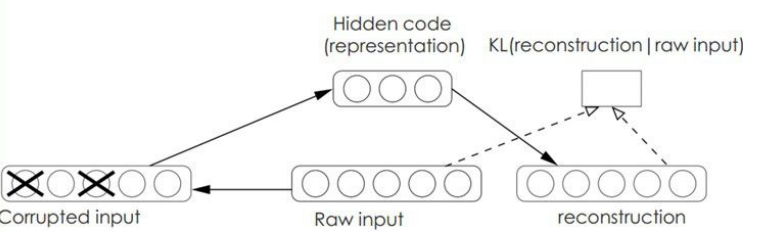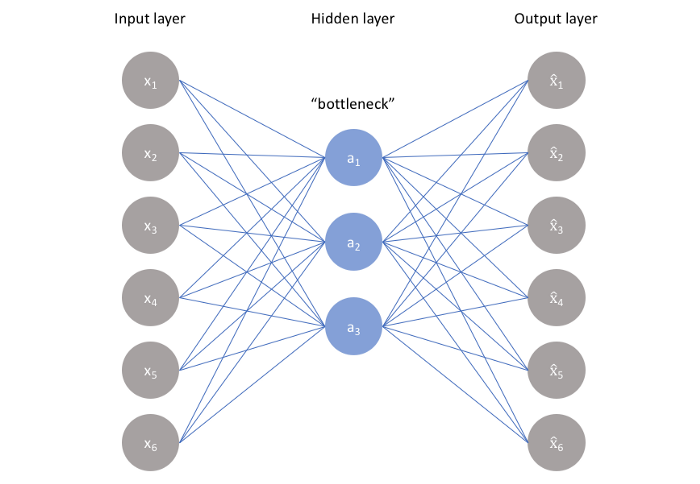# Autoencoder（自编码器）$J=\sum L(x,r)+\alpha\sum\limits_{i,j}W_{i,j}^2，其中W是权重矩阵$def fit(self, n_dimensions):
graph = tf.Graph()
with graph.as_default():

# 输入X
X = tf.placeholder(self.dtype, shape=(None, self.features.shape))

#初始化权重
encoder_weights = tf.Variable(tf.random_normal(shape=(self.features.shape, n_dimensions)))
encoder_bias = tf.Variable(tf.zeros(shape=[n_dimensions]))
decoder_weights = tf.Variable(tf.random_normal(shape=(n_dimensions, self.features.shape)))
decoder_bias = tf.Variable(tf.zeros(shape=[self.features.shape]))

# Encoder
encoding = tf.nn.sigmoid(tf.add(tf.matmul(X, encoder_weights), encoder_bias))

# Decoder
predicted_x = tf.nn.sigmoid(tf.add(tf.matmul(encoding, decoder_weights), decoder_bias))

#损失函数
cost = tf.reduce_mean(tf.pow(tf.subtract(predicted_x, X), 2))

with tf.Session(graph=graph) as session:
# Initialize global variables
session.run(tf.global_variables_initializer())

for batch_x in batch_generator(self.features):
self.encoder['weights'], self.encoder['bias'], _ = session.run([encoder_weights, encoder_bias, optimizer],
feed_dict={X: batch_x})
def reduce(self):
return np.add(np.matmul(self.features, self.encoder['weights']), self.encoder['bias'])